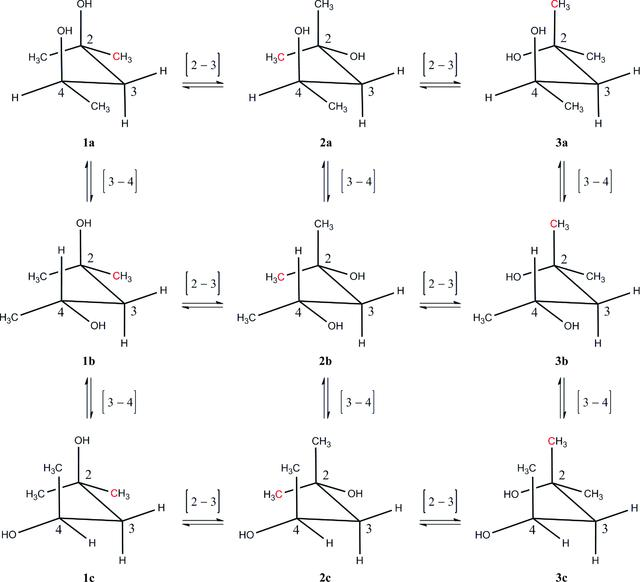disable zoom     view article Figure 2 The conformers of MPD studied by quantum-chemical calculations. Each conformer is denoted by a two-symbol code (number and letter) that approximately represents the torsion angles (ψ1, ψ2) of the initial conformation used in the calculation. The numbers 1, 2 and 3 correspond to ψ1 = 180, 300 and 60°, respectively; the letters a, b and c correspond to ψ2 = 180, 60 and 300°, respectively. [For example, 1a is the (180°, 180°) conformer shown in Fig. 1.] To interconvert two adjacent structures, a rotation is performed about the carbon–carbon bond given in square brackets. For clarity, only C2, C3 and C4 are numbered; C1 is shown in red, while CM and C5 (which is adjacent to C4) are shown in black.BIOLOGICALCRYSTALLOGRAPHY
ISSN: 1399-0047
Volume 71| Part 3| February 2015| Pages 427-441
Follow Acta Cryst. D